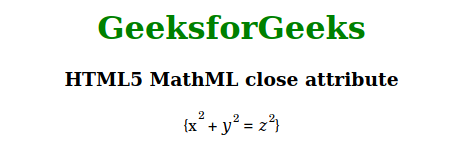Related Articles

# HTML5 MathML close Attribute

• Last Updated : 20 Nov, 2020

This attribute holds the closing parentheses of any equation the same as the open attribute. This attribute is accepted by the <mfenched> tag.

Syntax:

```<element close="parentheses">
```

Attribute value:

• Parentheses: It holds the parentheses which will be used to close any equation.

Example: Below example illustrates the close attribute in the HTML5 MathML.

## HTML

 ` ``<``html``> `` ` `<``body``> ``    ``<``center``> ``        ``<``h1` `style``=``"color:green"``> ``            ``GeeksforGeeks ``        `` ``         ` `        ``<``h3``>HTML5 MathML close attribute ``         ` `        ``<``math``> ``            ``<``mfenced` `open``=``"{"` `close``=``"}"` `separators``=``""``> ``                ``<``mrow``> ``                    ``<``msup``> ``                        ``<``mi``>x ``                        ``<``mn``>2 ``                    `` ``                    ``<``mo``>+ ``                    ``<``msup``> ``                        ``<``mi``>y ``                        ``<``mn``>2 ``                    `` ``                    ``<``mo``>= ``                    ``<``msup``> ``                        ``<``mi``>z ``                        ``<``mn``>2 ``                    `` ``                `` ``            `` ``        `` ``    `` `` `` ` ` `

Output:Supported browsers: The browser supported by HTML5 MathML close attribute are listed below.

• Firefox

Attention reader! Don’t stop learning now. Get hold of all the important Comcompetitivepetitve Programming concepts with the Web Design for Beginners | HTML  course.

My Personal Notes arrow_drop_up